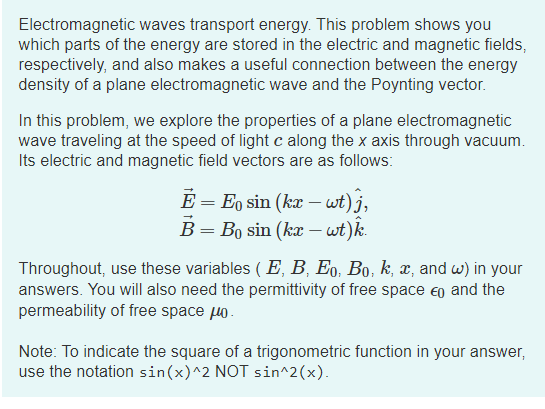# Problem: What is the instantaneous energy density UE (t) in the electric field of the wave?Give your answer in terms of some or all of the variables in E = E0 sin (kx-wt).

###### FREE Expert Solution

$\overline{){{\mathbf{U}}}_{{\mathbf{E}}}{\mathbf{\left(}}{\mathbf{t}}{\mathbf{\right)}}{\mathbf{=}}\frac{\mathbf{1}}{\mathbf{2}}{{\mathbf{\epsilon }}}_{{\mathbf{0}}}{{\mathbf{E}}}^{{\mathbf{2}}}}$###### Problem DetailsWhat is the instantaneous energy density UE (t) in the electric field of the wave?
Give your answer in terms of some or all of the variables in E = E0 sin (kx-wt).